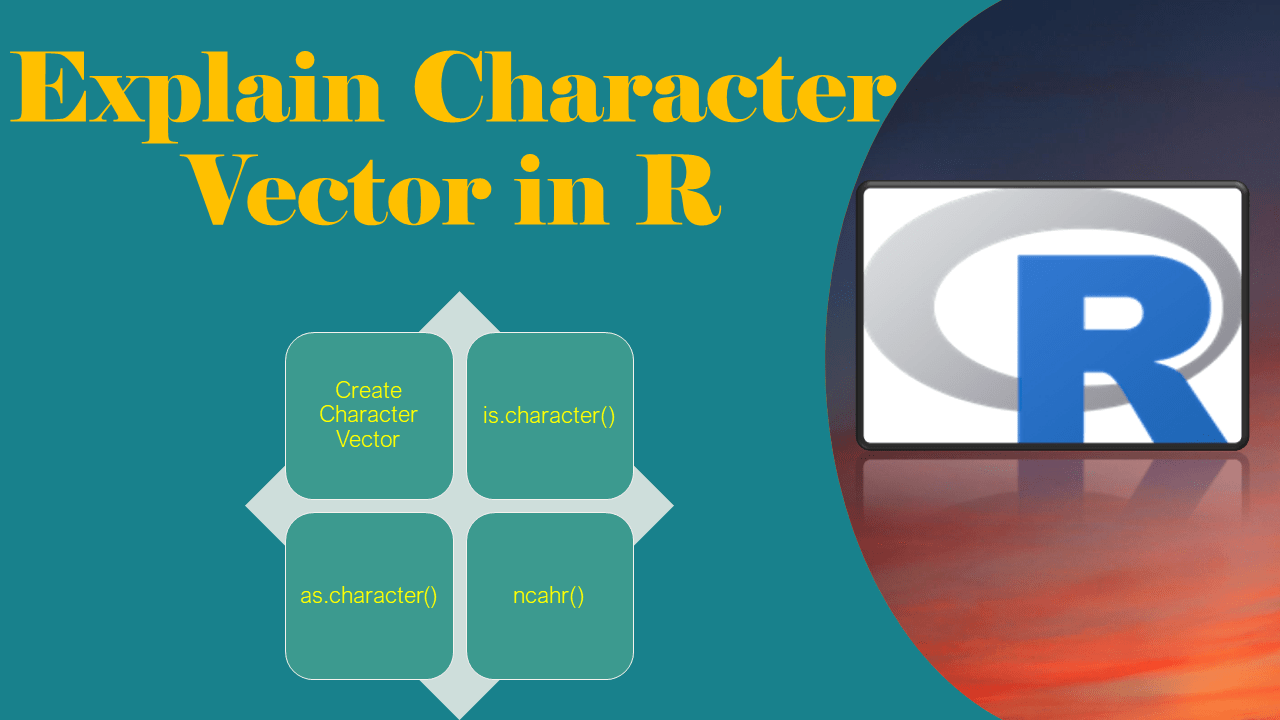# Explain Character Vector in R?

Character Vector in R is a vector of a type `character` that is used to store strings and NA values. In this article, I will explain what is a character vector, how to use it with methods like `character()`, `as.character()` and `is.character()` functions.

What is a Character Vector in R?

A vector where each element has only alphabet characters (a-z or A-Z) of any size is a character vector. For example,` c('ABC','abc','AbC','12AB')` is a character vector of length 3. One can create a character vector using c() or character() functions.

Following are the most used methods of character vector in R and their usage.

Character vector can have the following values

• A string of any length. for example `a`, `ABC`, `abc`, `AbC`
• Empty String “”
• NA

## 1. character()

`character()` function is used to create a character vector in R of a specified length. All elements of the R vector will contain the empty string “”. By default, not using a length creates an empty vector.

Following is the syntax of the `character()` vector.

``````
# Syntax of character
character(length = 0)
``````

The following example initializes a vector with 5 empty strings.

``````
# Usage of character()
v <- character(4)
print(v)
length(v)

# Output
# "" "" "" "" ""
# 5
``````

## 2. Create Character Vector

To create a vector use `c()` function and to create a character vector use string elements as values. Once the vector is created, check the type of the vector using `typeof()` function.

``````
# Create vector
v <- c('a','ABC','bc','A',NA)
print(v)
typeof(v)

# Output
# "a"   "ABC" "bc"  "A"   NA
# "character"
``````

## 3. is.character()

To check if the vector is of type character use `is.character()` function. This function takes a vector as an argument and returns TRUE for a character vector otherwise FALSE.

``````
# Check if vector is character type
is.character(v)

# Output
# TRUE

# Numeric vector
n <- c(1,2,3)
is.character(n)

# Output
# FALSE
``````

## 4. as.character()

`as.character()` function is used to convert the vector to the character type. This function takes the values of non-character and creates a character vector.

``````
# Convert elements to character vector
v <- as.character(1,2,3)
typeof(v)

# Output
# "character"
``````

## 5. ncahr()

The `nchar()` function is used to get the length of each element of the character vector in R. For the NA element it returns NA as length.

``````
# Get length of each element.
v <- c('a','ABC','bc','A',NA)
nchar(v)

# Output
#  1  3  2  1 NA
``````

## 6. Equal Condition

When you check if a value is equal in a vector by an equal condition, it returns a logical vector representing TRUE if a value is equal otherwise represents FALSE.

``````
res <- v == 'a'
print(res)

# Output
  TRUE FALSE FALSE FALSE    NA
``````

## 7. Conclusion

In this article, I have explained what is character vector in R, character functions, and how to use them. character() function initialized character vector with empty string. is.character() check if a vector is a character type. as.character() returns a character vector for non char elements. You can find the complete example from this article at Github R Programming Examples Project.

## References

### Naveen (NNK)

Naveen (NNK) is a Data Engineer with 20+ years of experience in transforming data into actionable insights. Over the years, He has honed his expertise in designing, implementing, and maintaining data pipelines with frameworks like Apache Spark, PySpark, Pandas, R, Hive and Machine Learning. Naveen journey in the field of data engineering has been a continuous learning, innovation, and a strong commitment to data integrity. In this blog, he shares his experiences with the data as he come across. Follow Naveen @ @ LinkedIn